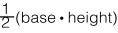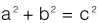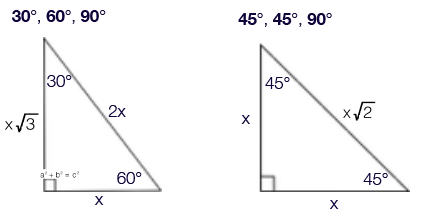# Geometry, For GMAT Notes | Study Quantitative Reasoning for GMAT - GMAT

## GMAT: Geometry, For GMAT Notes | Study Quantitative Reasoning for GMAT - GMAT

The document Geometry, For GMAT Notes | Study Quantitative Reasoning for GMAT - GMAT is a part of the GMAT Course Quantitative Reasoning for GMAT.
All you need of GMAT at this link: GMAT

GEOMETRY

WHY you need to know Geometry:

1. 4- 9 Geometry questions show up on the GMAT. 2. Geometry is based on a series of simple formulas. Knowing the formulas makes the questions easy to answer.
Note: If a Problem Solving question includes a drawing, then that drawing is usually to scale and can be used for estimation purposes (unless the question states that the ﬁgure is NOT drawn to scale). Note: If a Data Sufﬁciency question includes a drawing, then you should NOT TRUST IT. Some DS drawings are completely off; as such, they can not be used for estimation purposes. You can trust whatever numbers or descriptions you are given, but be suspicious of any picture that comes with a DS question.

Lines
Questions involving straight lines will likely include one or more of the following rules: 1. Lines add up to 180° 2. When lines criss-cross, opposite angles equal one another 3. Parallel lines, when crossed by a third line, will have the same set of 2 angles show up 4 times 4. Perpendicular lines form 90° angles at their meeting point

Triangles

Triangle questions will involve major rules and sometimes minor rules. Be on the lookout for each of these speciﬁc ideas on the GMAT: Major Rules:

Major Rules:

1. Triangles add up to 180°

2. Area =3. Pythagorean Theorem:4. Common Pythagorean triples:
• 3, 4, 5
• 5, 12, 13
5. Multiples of those triples for example:
• 9, 12, 15
• 50, 120, 130
6. 30°, 60°, 90° Triangle
7. 45°, 45°, 90° TriangleSometimes the following concepts show up; not every one will, but if they show, then you need to be ready for them.

Minor Rules:

1. Isosceles Triangles – 2 sides and 2 corresponding angles are equal
2. Equilateral Triangles – 3 sides are equal, all angles are 60 degrees
3. Hidden Right Triangles – in squares or equilateral triangles
4. Triangle Inequality Theorem – with 2 sides, you can ﬁgure out the minimum length of the third side (greater than the difference of the numbers) and the maximum (less than the sum of the numbers)

The document Geometry, For GMAT Notes | Study Quantitative Reasoning for GMAT - GMAT is a part of the GMAT Course Quantitative Reasoning for GMAT.
All you need of GMAT at this link: GMATUse Code STAYHOME200 and get INR 200 additional OFF

## Quantitative Reasoning for GMAT

75 videos|87 docs|156 tests

Track your progress, build streaks, highlight & save important lessons and more!

,

,

,

,

,

,

,

,

,

,

,

,

,

,

,

,

,

,

,

,

,

,

,

,

;